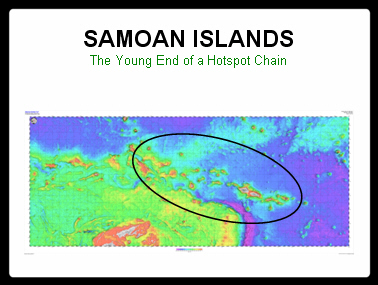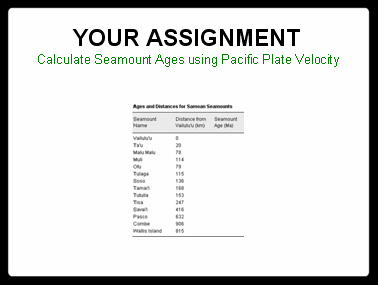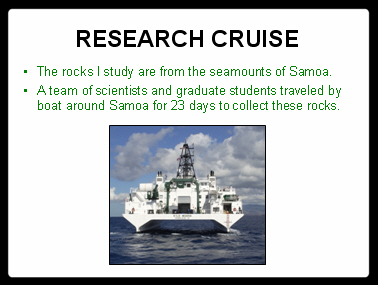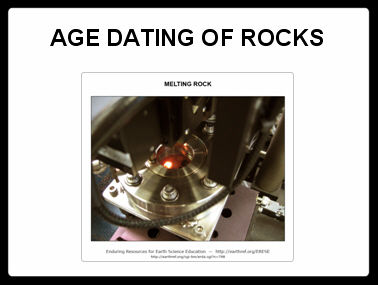# Hotspot Lesson: Samoan Hotspot

This material is replicated on a number of sites as part of the SERC Pedagogic Service Project

## Summary

This lesson makes comparisons between Hawaii and Samoa in an attempt to determine the volcanic origin of Samoa. The activity allows the students to calculate age estimates for the islands and seamounts of Samoa, assuming they were formed by the Pacific Plate traveling over a stationary hotspot located underneath Samoa. The students will use the Pacific plate velocity calculated using the Hawaiian data in one of the earlier hotspot classroom activities. It is assumed that both the Hawaiian and Samoan hotspots are fixed and are located underneath one-and-the-same tectonic plate.Used this activity? Share your experiences and modifications

## Learning Goals

• Test the hypothesis that the Samoan island and seamount chain was formed by a hotspot.
• Calculate the island/seamount ages using plate velocity data.
• ## Context for Use

This activity was designed for use in a high school earth science class but is also appropriate for introductory level college geoscience courses. The goal of this lesson is for the students to formulate hypotheses based on evidence from Samoa and make predictions based on their hypotheses. Lesson duration: Approximately 20 minutes.

## Description and Teaching Materials

This lesson begins with an introduction to Hotspots Chains using the Hawaiian Volcanic chain. The Samoan Islands are then introduced and a comparison drawn to the Hawaiian chain. This introduction can be given using the Samoa Introductory Lecture PowerPoint.

The activity is motivated by two questions:

• Is Samoa the result of a mantle plume, like Hawaii?
• Are there problems with the data?
• The instructor then divides the students into groups and assigns each group two seamounts or islands. The students calculate the age of the seamount using plate velocities from the previous activity on Hotspot Theory and Plate Velocities or the accepted velocity of 7 cm/year. The Samoa Activity Sheet walks them through the assignment. The Samoa Activity Instructions provides the data and template for the students to carry out the activity.The activity concludes with a whole class discussion. The students share their data and create an integrated data table. This data set can later be compared to actual age information calculated from Samoan samples given in Samoan Calculated Age Table. The students can then return to the motivating questions and discuss the origin of the Samona islands.

The Samoa Data Collection PowerPoint can be used to give students an idea of what kind of effort goes into doing research on island chains and seamounts.• This lecture makes comparisons between Hawaii and Samoa in an attempt to determine the volcanic origin of Samoa. The goal of this lesson is for the students to formulate hypotheses based on evidence from Samoa and make predictions based on their hypotheses. Download ...
• This activity allows the students to calculate age estimates for the islands and seamounts of Samoa, assuming they were formed by the Pacific Plate traveling over a stationary hotspot. Download ...
• This table provides the calculated ages for the Samoan islands and seamounts using a Pacific Plate velocity of 7 cm/year. This table can be used as a guideline for the approximate ages the students will calculate since a different plate velocity may be used in the calculations. Download ...
• This is the instruction sheet for the Samoa Activity. This document provides the data and equations that will be used to calculate the age of the Samoan islands and seamounts based on their distance from the currently active Vailulu'u volcano (likely location of the Samoan mantle plume). Download ...
• This additional lecture describes how science is conducted, from collecting samples, to processing the material and determining the results. This presentation is a summary of what Jamie does as a masters student at Scripps Institution of Oceanography. The goal of this lesson is to introduce students to some aspect of science that they may not have previously been familiar with. Download ...
• ## Teaching Notes and Tips

It is important to note that this is not entirely correct to use the velocity calculated using Hawaii data for Samoa because Hawaii is located far to the north of Samoa. Since the earth is spherical, latitude needs to be taken into account in determine angular rotations but for the scope of the class it was necessary to assume that the same velocity could be use.

Remember that the student values will not match those in the published data table if they use a velocity different that 7 cm/year.

## Assessment

Group participation and class participation can be assessed as well completion of the activity sheet.

This lesson ends with a Take Home Examine. This exam asks the students to compare two data sets of age data from Samoa. One graph is composed of ages collected prior to the data Jamie collected and the other graph including the new results. This exam evaluates the students understanding of some critical points from the weeks lectures.